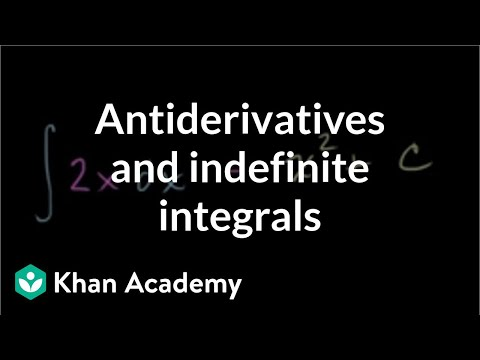### Calculus: Indefinite and definite integrals

Free Closed [?]Indefinite integral as anti-derivative. Definite integral as area under a curve. Integration by parts. U-substitution. Trig substitution.
Antiderivatives and indefinite integrals. Indefinite integrals of x raised to a power. Antiderivative of hairier expression. Basic trig and exponential antiderivatives. Antiderivative of x^-1. Simple Riemann approximation using rectangles. Generalizing a left Riemann sum with equally spaced rectangles. Rectangular and trapezoidal Riemann approximations. Trapezoidal approximation of area under curve. Riemann sums and integrals. Deriving integration by parts formula. Antiderivative of xcosx using integration by parts. Integral of ln x. Integration by parts twice for antiderivative of (x^2)(e^x). Integration by parts of (e^x)(cos x). U-substitution. U-substitution example 2. U-substitution Example 3. U-substitution with ln(x). Doing u-substitution twice (second time with w). U-substitution and back substitution. U-substitution with definite integral. (2^ln x)/x Antiderivative Example. Another u-substitution example. Riemann sums and integrals. Intuition for Second Fundamental Theorem of Calculus. Evaluating simple definite integral. Definite integrals and negative area. Area between curves. Area between curves with multiple boundaries. Challenging definite integration. Introduction to definite integrals. Definite integrals (part II). Definite Integrals (area under a curve) (part III). Definite Integrals (part 4). Definite Integrals (part 5). Definite integral with substitution. Introduction to trig substitution. Another substitution with x=sin (theta). Integrals: Trig Substitution 1. Trig and U substitution together (part 1). Trig and U substitution together (part 2). Trig substitution with tangent. Integrals: Trig Substitution 2. Integrals: Trig Substitution 3 (long problem). Fundamental theorem of calculus. Applying the fundamental theorem of calculus. Swapping the bounds for definite integral. Both bounds being a function of x. Proof of Fundamental Theorem of Calculus. Connecting the first and second fundamental theorems of calculus. Introduction to improper integrals. Improper integral with two infinite bounds. Divergent improper integral. Antiderivatives and indefinite integrals. Indefinite integrals of x raised to a power. Antiderivative of hairier expression. Basic trig and exponential antiderivatives. Antiderivative of x^-1. Simple Riemann approximation using rectangles. Generalizing a left Riemann sum with equally spaced rectangles. Rectangular and trapezoidal Riemann approximations. Trapezoidal approximation of area under curve. Riemann sums and integrals. Deriving integration by parts formula. Antiderivative of xcosx using integration by parts. Integral of ln x. Integration by parts twice for antiderivative of (x^2)(e^x). Integration by parts of (e^x)(cos x). U-substitution. U-substitution example 2. U-substitution Example 3. U-substitution with ln(x). Doing u-substitution twice (second time with w). U-substitution and back substitution. U-substitution with definite integral. (2^ln x)/x Antiderivative Example. Another u-substitution example. Riemann sums and integrals. Intuition for Second Fundamental Theorem of Calculus. Evaluating simple definite integral. Definite integrals and negative area. Area between curves. Area between curves with multiple boundaries. Challenging definite integration. Introduction to definite integrals. Definite integrals (part II). Definite Integrals (area under a curve) (part III). Definite Integrals (part 4). Definite Integrals (part 5). Definite integral with substitution. Introduction to trig substitution. Another substitution with x=sin (theta). Integrals: Trig Substitution 1. Trig and U substitution together (part 1). Trig and U substitution together (part 2). Trig substitution with tangent. Integrals: Trig Substitution 2. Integrals: Trig Substitution 3 (long problem). Fundamental theorem of calculus. Applying the fundamental theorem of calculus. Swapping the bounds for definite integral. Both bounds being a function of x. Proof of Fundamental Theorem of Calculus. Connecting the first and second fundamental theorems of calculus. Introduction to improper integrals. Improper integral with two infinite bounds. Divergent improper integral.

Categories:

# Reviews

-- no reviews yet -- make the first review

# Alternatives All alternatives to all Khan Academy courses

-- no alternatives found for the course --
If you know any alternatives, please let us know.

# Prerequisites

-- no prerequsites found for the course --
If you can suggest any prerequisite, please let us know.

# Paths

No Paths inclusing the course. You can build and share a path with this course included.

# Certification Exams

-- there are no exams to get certification after this course --
If your company does certification for those who completed this course then register your company as certification vendor and add your exams to the Exams Directory.

# Similar courses

Courses related to the course subject

### Calculus I (Differential Calculus)

Videos on a first course in calculus (Differential Calculus).

### Calculus II (Integral Calculus)

Videos on a second course in calculus (Integral Calculus).

### Calculus One

Calculus One is a first introduction to differential and integral calculus, emphasizing engaging examples from everyday life.

Details:

### Calculus: AP Calculus practice questions

Sample questions from the A.P. Calculus AB and BC exams (both multiple choice and free answer). 2011 Calculus AB Free Response #1a…

### Calculus: Derivative applications

Minima, maxima, and critical points. Rates of change. Optimization. Rates of change. L'Hopital's rule. Mean value theo…

### Calculus: Divergence theorem

Divergence theorem intuition. Divergence theorem examples and proofs. Types of regions in 3D. 3-D Divergence Theorem Intuition.

### Calculus: Double and triple integrals

Volume under a surface with double integrals. Triple integrals as well. Double Integral 1. Double Integrals 2. Double Integrals 3.

### Calculus: Indefinite and definite integrals

Indefinite integral as anti-derivative. Definite integral as area under a curve. Integration by parts. U-substitution. Trig su…

### Calculus: Limits

Limit introduction, squeeze theorem, and epsilon-delta definition of limits. Introduction to limits. Limit at a point of discontin…

### Calculus: Line integrals and Green's theorem

Line integral of scalar and vector-valued functions. Green's theorem and 2-D divergence theorem. Introduction to the Line In…

Let us know when you did the course Calculus: Indefinite and definite integrals.

 Started on: Completed on: Your grade (if any):Comments:Add the course Calculus: Indefinite and definite integrals to My Personal Education Path.

 Start the course on: Duration of study: 1 Week 2 Weeks 3 Weeks 4 Weeks 5 Weeks 6 Weeks 7 Weeks 8 Weeks 10 Weeks 12 Weeks 16 Weeks Notes: